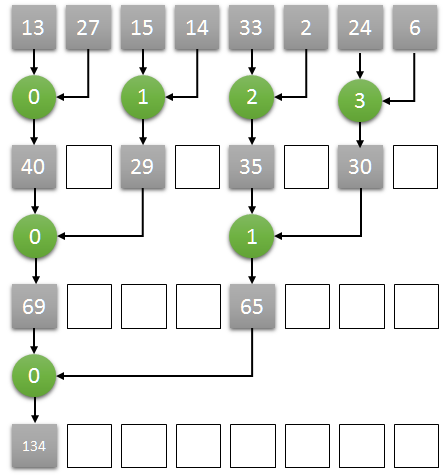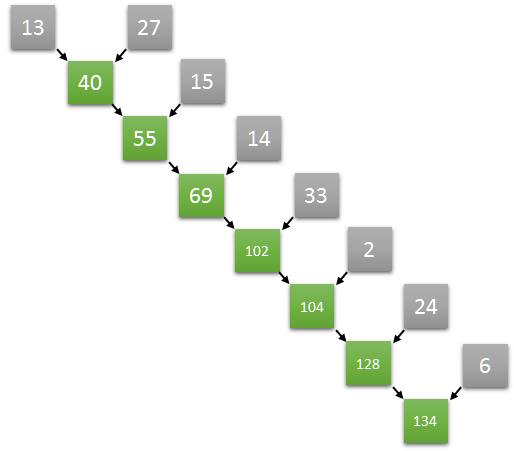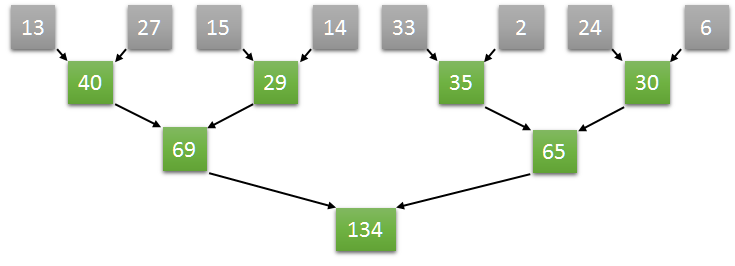Tech Trusted

# Implementing Parallel Reduction in CUDAReduction operations are those that reduce a collection of values to a single value. In this post, I will share how to implement parallel reduction operations using CUDA.

## Sequential Sum

Compute the sum of all elements of an array is an excellent example of reduction operation. The sum of an array which values are 13, 27, 15, 14, 33, 2, 24, and 6 is 134.

The interesting question is: How would you compute it? Probably your first answer would be doing something like this (((((((13+27)+15)+14)+33)+2)+24)+6). Am I right?The problem with this approach is that it is impossible to parallelize. Why? Each step depends on the result of the previous one.

## Parallel Sum

Adding values is an associative operation. So, we can  try something like this ((13+27)+(15+14))+((33+2)+(24+6))This way is much better because now we can execute it in parallel!

## CUDA

Let’s figure out how to do it using CUDA.Here is the main idea:

• Assuming N as the number of the elements in an array, we start N/2 threads, one thread for each two elements
• Each thread computes the sum of the corresponding two elements, storing the result at the position of the first one.
• Iteratively, each step:
• the number of threads halved (for example, starting with 4, then 2, then 1)
• doubles the step size between the corresponding two elements (starting with 1, then 2, then 4)
• after some iterations, the reduction result will be stored in the first element of the array.

Let’s code

```#include "cuda_runtime.h"
#include "device_launch_parameters.h"

#include <iostream>
#include <numeric>
using namespace std;

__global__ void sum(int* input)
{

auto step_size = 1;

{
if (tid < number_of_threads) // still alive?
{
const auto fst = tid * step_size * 2;
const auto snd = fst + step_size;
input[fst] += input[snd];
}

step_size <<= 1;
}
}

int main()
{
const auto count = 8;
const int size = count * sizeof(int);
int h[] = {13, 27, 15, 14, 33, 2, 24, 6};

int* d;

cudaMalloc(&d, size);
cudaMemcpy(d, h, size, cudaMemcpyHostToDevice);

sum <<<1, count / 2 >>>(d);

int result;
cudaMemcpy(&result, d, sizeof(int), cudaMemcpyDeviceToHost);

cout << "Sum is " << result << endl;

getchar();

cudaFree(d);
delete[] h;

return 0;
}
```

## Time to action

In this post, we implemented a primary example of parallel reduction operation in CUDA. But, the pattern we adopted can be used in more sophisticated scenarios.

I strongly recommend you to try to implement other reduction operations (like discovering the max/min values of an array). Now, it would be easy. Right?

Compartilhe este insight:

### 6 respostas

1. Luís Barbosa disse:

Hi Elemar,

what do you think about projects like Hybridizer and ManagedCuda?

I’ve been testing ALEA.. It is “nice”

2. Shivani Shinde disse:

What do following lines do?
step_size <>= 1;
Or partiularly, what is functioning of “<>==”?

1. Rahul disse:

The line is:
step_size<<=1
It doubles the step_size.

3. Liangzhi Tang disse:

How to sum using multiple blocks?

4. me disse:

hi, what happens when the size of the array is not a power of two? Im trying to do it with an array of 784 elemnts but it does no sum well, do you have any ideas? thanks in advance## Elemar Júnior

Sou fundador e CEO da EximiaCo e atuo como tech trusted advisor ajudando diversas empresas a gerar mais resultados através da tecnologia.## Elemar Júnior

Sou fundador e CEO da EximiaCo e atuo como tech trusted advisor ajudando diversas empresas a gerar mais resultados através da tecnologia.

Mais insights para o seu negócio

Veja mais alguns estudos e reflexões que podem gerar alguns insights para o seu negócio:

## [QUESTION] Which version of this function is faster? Why?

Our goal is to fill a two-dimensional array with 1’s. using BenchmarkDotNet.Attributes; using BenchmarkDotNet.Running; namespace ToArrays { public class Program...

## Getting started with CUDA (using VS2017)

If you are interested in performance, you need to know more about CUDA. From the official website: CUDA® is a...

## Modelagem de Documentos no Mundo Real: Configuration Documents

Frequentemente precisamos fazer referência para outros documentos e isso é natural. Entretanto, há cenários onde o documento que queremos referenciar...

## QUESTION: Why does not this program quit when compiled in Release mode?

The following works as expected when building in Debug – the execution is done after three seconds. But, for some...

## [QUESTION] Here are Common Mistakes When Working with Enumerables. Can you see it?

The following code contains some of the most common mistakes I have been seeing when reviewing code that deals with...